SSC CGL Previous Year Questions: Profit & Loss - 2

# SSC CGL Previous Year Questions: Profit & Loss - 2 - SSC CGL

Test Description

## 30 Questions MCQ Test SSC CGL (Tier - 1) - Previous Year Papers (Topic Wise) - SSC CGL Previous Year Questions: Profit & Loss - 2

SSC CGL Previous Year Questions: Profit & Loss - 2 for SSC CGL 2023 is part of SSC CGL (Tier - 1) - Previous Year Papers (Topic Wise) preparation. The SSC CGL Previous Year Questions: Profit & Loss - 2 questions and answers have been prepared according to the SSC CGL exam syllabus.The SSC CGL Previous Year Questions: Profit & Loss - 2 MCQs are made for SSC CGL 2023 Exam. Find important definitions, questions, notes, meanings, examples, exercises, MCQs and online tests for SSC CGL Previous Year Questions: Profit & Loss - 2 below.
Solutions of SSC CGL Previous Year Questions: Profit & Loss - 2 questions in English are available as part of our SSC CGL (Tier - 1) - Previous Year Papers (Topic Wise) for SSC CGL & SSC CGL Previous Year Questions: Profit & Loss - 2 solutions in Hindi for SSC CGL (Tier - 1) - Previous Year Papers (Topic Wise) course. Download more important topics, notes, lectures and mock test series for SSC CGL Exam by signing up for free. Attempt SSC CGL Previous Year Questions: Profit & Loss - 2 | 30 questions in 30 minutes | Mock test for SSC CGL preparation | Free important questions MCQ to study SSC CGL (Tier - 1) - Previous Year Papers (Topic Wise) for SSC CGL Exam | Download free PDF with solutions
 1 Crore+ students have signed up on EduRev. Have you?
SSC CGL Previous Year Questions: Profit & Loss - 2 - Question 1

### The price of an article is cut by 33%, to restore to its original value, the new price must be increased by    (SSC CHSL 2017)

Detailed Solution for SSC CGL Previous Year Questions: Profit & Loss - 2 - Question 1

Let the price of the article = ₹ 100
New Price = 100 – 33 = 67
Therefore the new price must be increased by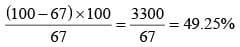SSC CGL Previous Year Questions: Profit & Loss - 2 - Question 2

### Marked price of an item is Rs 500. On purchase of 2 items discount is 8%, on purchase of 3 items discount is 16%. Radha buys 5 items, what is the effective discount?    (SSC CHSL 2017)

Detailed Solution for SSC CGL Previous Year Questions: Profit & Loss - 2 - Question 2

Marked price of each item = 500
No. of items = 5
∴ Total marked price = 500 × 5 = 2500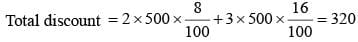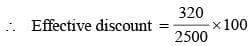= 12.8%

SSC CGL Previous Year Questions: Profit & Loss - 2 - Question 3

### A person bought pens at 25 for a rupee and sold at 15 for a rupee. What is his profit percentage?    (SSC CGL 2017)

Detailed Solution for SSC CGL Previous Year Questions: Profit & Loss - 2 - Question 3

According to the question,
C.P of a pen = 1/25
S. P of a pen = 1/15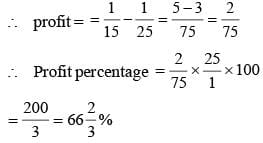SSC CGL Previous Year Questions: Profit & Loss - 2 - Question 4

The marked price of an article is 20% more than its cost price. If 5% discount is given on the maked price, then what is the profit percentage?    (SSC CGL 2017)

Detailed Solution for SSC CGL Previous Year Questions: Profit & Loss - 2 - Question 4

Let C.P of an article = 100
∴ M.P of an article = 120
After discount 5% on marked price, then
S.P of an article = 120 × 0.95 = 114.
∴ Profit = 114 – 100 = 14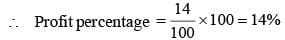SSC CGL Previous Year Questions: Profit & Loss - 2 - Question 5

By selling 175 pineapples, the gain i s equal to the selling price of 50 pineapples. What is the gain percentage?    (SSC CGL 2017)

Detailed Solution for SSC CGL Previous Year Questions: Profit & Loss - 2 - Question 5

Let S.P. of each pineapple = Re 1
∴ Gain = 50, SP = 175
∴ CP = (175 – 50) = 125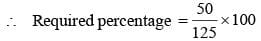= 40%

SSC CGL Previous Year Questions: Profit & Loss - 2 - Question 6

The marked price of a sofa set is ₹ 4800 which is sold at ₹ 3672 at two successive discounts. If the first discount is 10%, then what will be the second discount (in%)?    (SSC CGL 2017)

Detailed Solution for SSC CGL Previous Year Questions: Profit & Loss - 2 - Question 6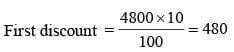Price after it = 4800 – 480 = 4320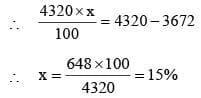∴ Second discount = 15%

SSC CGL Previous Year Questions: Profit & Loss - 2 - Question 7

If the price of pen decreases by 20%, then a man can buy 10 more pens for ₹ 100. What is the new price  (in ₹) of each pen?     (SSC CGL 2017)

Detailed Solution for SSC CGL Previous Year Questions: Profit & Loss - 2 - Question 7

Let the cost price of pen = x
∴ Number of pens he can purchase for ₹ 100 with the actual price of pen = 100/x
Number of pens he can purchase for ₹ 100 with the reduced price of pen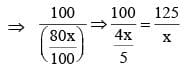According to question,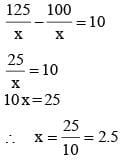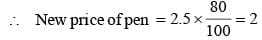SSC CGL Previous Year Questions: Profit & Loss - 2 - Question 8

After two successive discount of 20% and 35%, an article is sold for ₹ 50700. What is the marked price (in ₹) of the article?    (SSC CGL 2017)

Detailed Solution for SSC CGL Previous Year Questions: Profit & Loss - 2 - Question 8

Let the mark price be x.
After first discount, price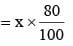after the second discount, price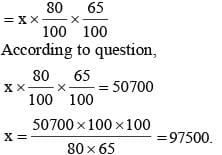∴ marked price of article is ₹ 97500.

SSC CGL Previous Year Questions: Profit & Loss - 2 - Question 9

For an article the profit is 170% of the cost price. If the cost price increases by  20% but the selling price remains same, then what is the new profit percentage?    (SSC CGL 2017)

Detailed Solution for SSC CGL Previous Year Questions: Profit & Loss - 2 - Question 9

Let cost price of an article = 100
∴ Profit = 170
∴ S.P. = 100 + 170 = 270
Now,
Cost price increased by 20%, then
Cost price = 120
S.P. = 270
∴ Profit = 270 – 120 = 150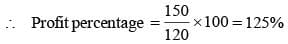SSC CGL Previous Year Questions: Profit & Loss - 2 - Question 10

After a discount of 3 4% an article is s old for ₹ 3168. What is the marked price (in ₹) of the article?    (SSC CGL 2017)

Detailed Solution for SSC CGL Previous Year Questions: Profit & Loss - 2 - Question 10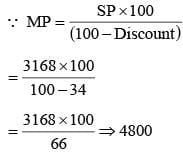SSC CGL Previous Year Questions: Profit & Loss - 2 - Question 11

A merchant purchase a wrist watch for ₹ 1,200 and fixes its list price in such a way that after allowing a discount of 10%, he earns a profit of 20%. The list price of the watch is    (SSC Sub Inspector 2016)

Detailed Solution for SSC CGL Previous Year Questions: Profit & Loss - 2 - Question 11

Marked price of the wrist watch = ₹ x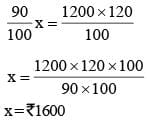SSC CGL Previous Year Questions: Profit & Loss - 2 - Question 12

By selling an article at 3/4 of selling price, a trader incurred a loss of 10%. The profit/loss percentage, when it is sold at the original selling prices, is  (SSC Sub Inspector 2016)

Detailed Solution for SSC CGL Previous Year Questions: Profit & Loss - 2 - Question 12

Let selling price be 100
Now selling price =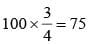Loss = 10%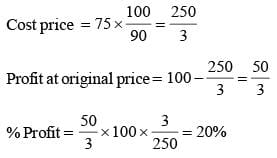SSC CGL Previous Year Questions: Profit & Loss - 2 - Question 13

A trader lists his article 20% above the cost price and allows a discount of 10% on cash payment. His gain percent is    (SSC Sub Inspector 2016)

Detailed Solution for SSC CGL Previous Year Questions: Profit & Loss - 2 - Question 13

Let C.P. = ₹ 100
Then, M.P. = ₹ 120
S.P. = 90% of ₹ 120 = ₹ 108
Gain = 8%

SSC CGL Previous Year Questions: Profit & Loss - 2 - Question 14

A man sells an article at 5% above the cost price. If he had bought it at 5% less than what he paid for it and sold it for ₹ 2 less, he would have gained 10%. The cost price of the article is    (SSC Sub Inspector 2016)

Detailed Solution for SSC CGL Previous Year Questions: Profit & Loss - 2 - Question 14

Let C.P. = x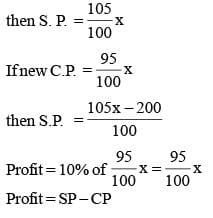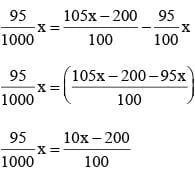95x = 100 x – 2000
– 5x = – 2000
x = ₹ 400
C.P. = ₹ 400

SSC CGL Previous Year Questions: Profit & Loss - 2 - Question 15

Loss of 20% on selling price is equal to x% loss in cost price. What is x?    (SSC CGL 2nd Sit. 2016)

Detailed Solution for SSC CGL Previous Year Questions: Profit & Loss - 2 - Question 15

Let  Selling Price = 100
Loss = 20%
Cost price = 120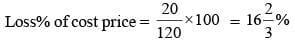SSC CGL Previous Year Questions: Profit & Loss - 2 - Question 16

A dealer marks a washing machine for ₹ 7500, and allows a discount of 6% on it. Find the selling price    (SSC CGL 2nd Sit. 2016)

Detailed Solution for SSC CGL Previous Year Questions: Profit & Loss - 2 - Question 16

Market Price = 7500, Discount = 6%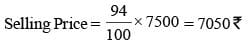SSC CGL Previous Year Questions: Profit & Loss - 2 - Question 17

If the ratio of cost price and selling price be 10 : 11, then the profit percentage is    (SSC CGL 2nd Sit. 2016)

Detailed Solution for SSC CGL Previous Year Questions: Profit & Loss - 2 - Question 17

Let CP = 10, SP = 11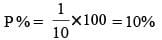SSC CGL Previous Year Questions: Profit & Loss - 2 - Question 18

The successive discount of 15%, 20% and 25% on an article is equivalent to the single discount of    (SSC CGL 1st Sit. 2016)

Detailed Solution for SSC CGL Previous Year Questions: Profit & Loss - 2 - Question 18

Successive discount = 15, 20, 25
net discount when, 15, 20 taken together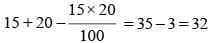Now taking 22 and 25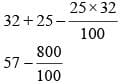57 – 8 = 49%

SSC CGL Previous Year Questions: Profit & Loss - 2 - Question 19

The marked price of a ceiling fan is ₹ 1200 and the shopkeeper allows a discount of 5 % on it. Then selling price of the fan is (SSC CGL 1st Sit. 2016)

Detailed Solution for SSC CGL Previous Year Questions: Profit & Loss - 2 - Question 19

Marked price = 1200, Discount % = 5%
Selling price = ?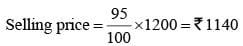SSC CGL Previous Year Questions: Profit & Loss - 2 - Question 20

The selling price of 6 bananas is equal to the cost price of 8 bananas. Then the percentage of profit is    (SSC CGL 1st Sit. 2016)

Detailed Solution for SSC CGL Previous Year Questions: Profit & Loss - 2 - Question 20

Let CP of 8 bananas = ₹ 8
CP of 6 bananas = ₹ 6
Sp of 6 bananas = ₹ 8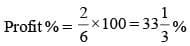SSC CGL Previous Year Questions: Profit & Loss - 2 - Question 21

If the successive discounts be 20%, 10% and 5%, then the single equivalent rate of discount is    (SSC CGL 1st Sit. 2016)

Detailed Solution for SSC CGL Previous Year Questions: Profit & Loss - 2 - Question 21

For 3 discount 20%, 10% and 5%
Now take 20% and 10%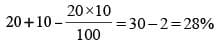Now take 28 % and 5%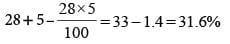SSC CGL Previous Year Questions: Profit & Loss - 2 - Question 22

An article which is marked ₹ 975 is sold for ₹ 897. The discount % is?    (SSC CGL 1st Sit. 2015)

Detailed Solution for SSC CGL Previous Year Questions: Profit & Loss - 2 - Question 22

Now, Let the discount be x%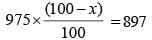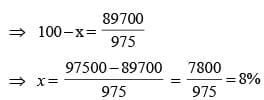SSC CGL Previous Year Questions: Profit & Loss - 2 - Question 23

Cost price of 100 books is equal to the selling price of 60 books. The gain or loss percentage will be:    (SSC CGL1st Sit. 2015)

Detailed Solution for SSC CGL Previous Year Questions: Profit & Loss - 2 - Question 23

Now according to question.
100 × CP = 60 × SP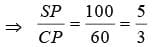Both sides subtract 1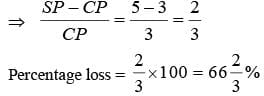SSC CGL Previous Year Questions: Profit & Loss - 2 - Question 24

Find a single discount equivalent to a discount series of 10%, 20% and 25%    (SSC CGL 1st Sit.  2015)

Detailed Solution for SSC CGL Previous Year Questions: Profit & Loss - 2 - Question 24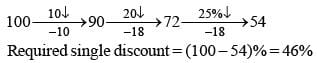SSC CGL Previous Year Questions: Profit & Loss - 2 - Question 25

The difference between successive discounts of 4 0% followed by 30% and 45% followed by 20% on the maked price of an article is ₹ 12. The marked price of the article is:    (SSC CGL 1st Sit. 2015)

Detailed Solution for SSC CGL Previous Year Questions: Profit & Loss - 2 - Question 25

1st successive discount final rate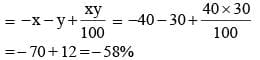2nd successive discount final rateLet mare price be MP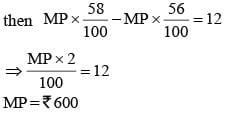SSC CGL Previous Year Questions: Profit & Loss - 2 - Question 26

A shopkeeper bought 30 kg of rice at the rate of ₹ 70 per kg and 20 kg of rice at the rate of ₹ 70.75 per kg. If he mixed the two brand of rice and sold the mixture at ₹ 80.50 per kg. his gain is    (SSC CGL 2nd Sit. 2015)

Detailed Solution for SSC CGL Previous Year Questions: Profit & Loss - 2 - Question 26

Solving by allegation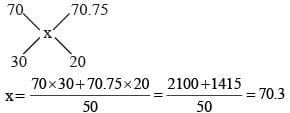Hence, cost price = 70.3 × 50 = ₹ 3515
Selling price = 80.5 × 50 = ₹ 4025
Required gain = 4025 – 3515 = 510

SSC CGL Previous Year Questions: Profit & Loss - 2 - Question 27

Allowing 20% and 15% successive discounts, the selling price of an article becomes ₹ 3,060; then the marked price will be            (SSC CGL 2nd Sit. 2015)

Detailed Solution for SSC CGL Previous Year Questions: Profit & Loss - 2 - Question 27

S.P. of an article = 20% and 15% successive discount × marked price of an article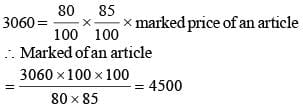SSC CGL Previous Year Questions: Profit & Loss - 2 - Question 28

The marked price of a watch was ₹ 720. A man bought the same for ₹ 550.80 after getting two successive discounts, the first being 10%. The second discount rate is    (SSC CGL 2nd Sit. 2015)

Detailed Solution for SSC CGL Previous Year Questions: Profit & Loss - 2 - Question 28

Let the second discount be x%.
Then (100 – x)% of 90% of 720 = 550.80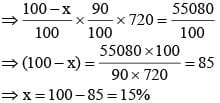SSC CGL Previous Year Questions: Profit & Loss - 2 - Question 29

10% discount and then 20% discount in succession is equivalent to total discount of    (SSC CGL 2nd Sit. 2015)

Detailed Solution for SSC CGL Previous Year Questions: Profit & Loss - 2 - Question 29

Successive discount can be given by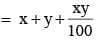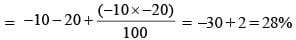Hence, the successive discount in equal to 28%

SSC CGL Previous Year Questions: Profit & Loss - 2 - Question 30

After allowing a discount of 20%, a radio is available for ₹ 1200. Its marked price was:    (SSC CHSL 2nd Sit. 2015)

Detailed Solution for SSC CGL Previous Year Questions: Profit & Loss - 2 - Question 30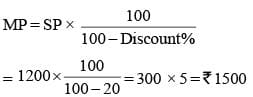## SSC CGL (Tier - 1) - Previous Year Papers (Topic Wise)

250 tests
Information about SSC CGL Previous Year Questions: Profit & Loss - 2 Page
In this test you can find the Exam questions for SSC CGL Previous Year Questions: Profit & Loss - 2 solved & explained in the simplest way possible. Besides giving Questions and answers for SSC CGL Previous Year Questions: Profit & Loss - 2, EduRev gives you an ample number of Online tests for practice

250 tests